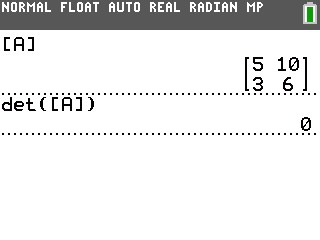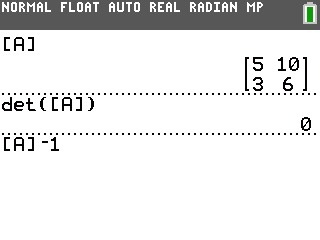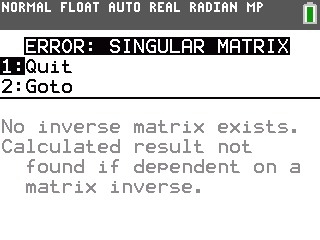# Knowledge Base

## Solution 34498: Causes of a Singular Matrix Error on the TI-83 Plus and TI-84 Plus Family of Graphing Calculators.

### What causes a Singular Matrix error message on the TI-83 Plus and TI-84 Plus family of graphing calculators?

The message "ERROR:SINGULAR MATRIX" may be the result of one of two possible things. The first reason is an attempt to take the inverse of a matrix for which the determinant is zero. A second possible cause for this error message is if the coordinates provided are only from a single cycle of the sine wave. A minimum of two points from two cycles of the sine wave are needed to generate an answer.

Example:

Matrix A, shown below, has a determinant of 0. The determinant is shown by using the det command.Here we attempt to find the inverse, shown below, of Matrix A.This results in the Singular Matrix error. This error also occurs if a calculation (typically a regression) produces a singular matrix.For more information on the algorithm used for calculating the Sine Regression please see Solution 11576: Algorithm Used for Calculating the Sine Regression.

Please see the TI-83 Plus and TI-84 Plus Family guidebooks for additional information.